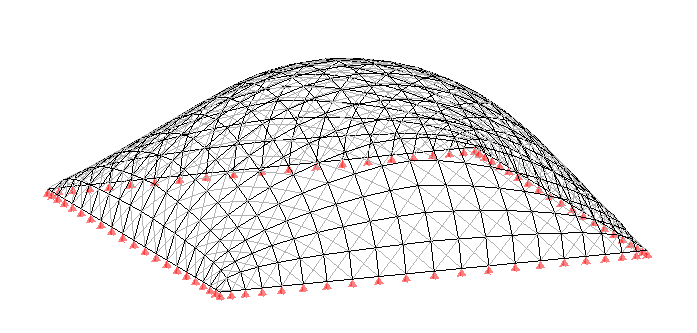One of the forms of pretensioning a membrane is to overpressure of the interior air. When a membrane is subjected to an air pressure has a certain curvature, this pressure causes the membrane a stress that is proportional to the curvature.

So, if it’s a membrane of cylindrical shape, with a certain radius of curvature R, T tension caused by the internal pressure P will be

`T = P x R`

This is in the direction of the curvature of the cylinder. The tension in the direction of the guideline of the cylinder is zero as there is no curvature. In case of a sphere (or part of a sphere) we see curvature in all the directions, therefore tension provoked by the internal pressure must decompose in two orthogonal directions giving a tension on the membrane.

`T = P x R / 2`

in any direction of the membrane. In case of other irregular shape, tension of the membrane produced by internal pressure will depend on the radius of curvature of the membrane in the direction that we study. In fact the stress depends on the radius of curvature R1 and the radius of curvature R2 in orthogonal directions to which we are studying. The equation is always supported

`P = T1 / R1 + T2 / R2`

It is easy to see that this equation is valid also for the two cases discussed above. In the first the radius of curvature R2 of the guideline of the cylinder is ∞, with what T2 / R2 = 0, whereas in the second case R1 = R2 and therefore T1 = T2 and consequently P = 2 x (T1 / R1).

Here we are dealing with the theme of pneumatic structures in initial state. But what happens when a pneumatic structure is subjected to an external action? For example, snow or wind. The membrane deforms due to these loads as the existing balance between internal pressure and tension of the membrane breaks.

However, the deformed pneumatic structure, more likely (the opposite would be a coincidence) is to modify the interior volume of the pneumatic structure. And here comes a new element to take into account: change of the internal pressure. This change can be important in some cases and determinant in others. From the Law of Boyle-Mariotte we know that at constant temperature, multiplication of volume and pressure of a gas stays constant. As the air is a gas, it follows this law. If the interior volume is modified of a pneumatic structure, the interior pressure is modified as well.

To implement this law in a structure pneumatic we must take into account what is the true internal pressure of the air. In general we usually define the pressure of a pneumatic structure as a value of overpressure. Namely the difference between the interior pressure and the exterior pressure. So when we say that the pressure of some structure pneumatic is of 300 Pa (30 kg/m²), we mean, in reality, that the internal pressure is 300 Pa higher than that of outside. In general, as we assume that the external pressure is atmospheric pressure (approx. 100 kPa), what we have in reality is an internal pressure of 100300 Pa. This is the value we use in our calculations when we want take into account the change of internal pressure due to the volume change.

Let us look at this with an example.Initial state

In the image above, we see a structure pneumatic in the initial state with an interior volume of 1456 m³ and an internal pressure of 300 Pa. Using WinTess we apply wind load of 120 km/h and find a balance with a certain deformation. However we can see how the interior volume current has changed to 1488 m³ due to the suction of the wind. This increase in volume has been due to decrease of the internal pressure. If we call the initial pressure as P0, we will have the final pressure Pf is

`Pf x 1488 = P0 x 1456 ; Pf = P0 x 1456 / 1488`

We must not make the mistake of thinking that P0 = 300 Pa and that, therefore, Pf = 293 Pa. But that we must use the actual values: P0 = 100300 Pa and Pf = 98143 Pa. This value is less than the atmospheric pressure and therefore the membrane is sucked inward, instead of being expanded by the internal pressure. The structure is not in balance!

The same thing, but in the opposite direction would be to assume a snow load. If we apply a snow load of 40 kg/m² on this same initial structure, as this loads greater than the internal pressure, the membrane will be getting lower to reach the ground. But be careful! When you decrease the volume, the internal pressure increases and therefore there will a moment the internal pressure will be able to bear the snow load.

If we use WinTess3 and activate the change of internal pressure by changing the volume, you’ll find that we find a balance around 1452 m³. It seems to be a value very close to the initial 1456 m³, but if we apply the above equations we will see that:

`Pf = P0 x 1456 / 1452 = 100576 Pa`

And this value means, with respect to atmospheric pressure outside, an internal pressure of 57.6 kg/m², able to withstand snow loads of 40 kg/m². The structure is supported.

Another thought, we’re not going to do here, is the take into account if the structure provides a system of sealing capable to make sure that the air inside remains constant while the pneumatic structure deforms. This is another topic!